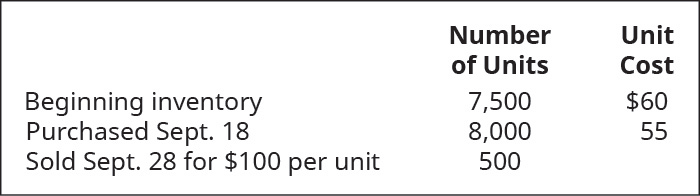# Use the first-in, first-out method (FIFO) cost allocation method, with perpetual inventory updating, to calculate (a) sales revenue, (b) cost of goods sold, and c) gross margin for B75 Company, considering the following transactions.FindFindarrow_forward

### Principles of Accounting Volume 1

19th Edition
OpenStax
Publisher: OpenStax College
ISBN: 9781947172685

#### Solutions

Chapter
SectionFindFindarrow_forward

### Principles of Accounting Volume 1

19th Edition
OpenStax
Publisher: OpenStax College
ISBN: 9781947172685
Chapter 10, Problem 5PB
Textbook Problem
12 views

## Use the first-in, first-out method (FIFO) cost allocation method, with perpetual inventory updating, to calculate (a) sales revenue, (b) cost of goods sold, and c) gross margin for B75 Company, considering the following transactions.To determine

Concept introduction:

Perpetual Inventory System: The perpetual inventory system maintains records and updates the inventory after each transaction. The inventory balance is updated after every transaction.

FIFO method: FIFO Stands for First in First Out. Under this method,the units purchased first are assumed to be issued first and cost of goods sold is calculated accordingly. The ending inventory in the method includes the latest purchasedunits.

Requirement-1:

To calculate:

Sales revenue.

### Explanation of Solution

 Number of Units Unit Rate Total
To determine

Concept introduction:

Perpetual Inventory System: The perpetual inventory system maintains records and updates the inventory after each transaction. The inventory balance is updated after every transaction.

FIFO method: FIFO Stands for First in First Out. Under this method, the units purchased first are assumed to be issued first and cost of goods sold is calculated accordingly. The ending inventory in the method includes the latest purchasedunits.

Requirement-2:

To calculate:

Cost of goods sold

To determine

Concept introduction:

Perpetual Inventory System: The perpetual inventory system maintains records and updates the inventory after each transaction. The inventory balance is updated after every transaction.

FIFO method: FIFO Stands for First in First Out. Under this method, the units purchased first are assumed to be issued first and cost of goods sold is calculated accordingly. The ending inventory in the method includes the latest purchasedunits.

Requirement-3:

To calculate:

Gross margin using FIFO perpetual inventory method.

### Still sussing out bartleby?

Check out a sample textbook solution.

See a sample solution

#### The Solution to Your Study Problems

Bartleby provides explanations to thousands of textbook problems written by our experts, many with advanced degrees!

Get Started